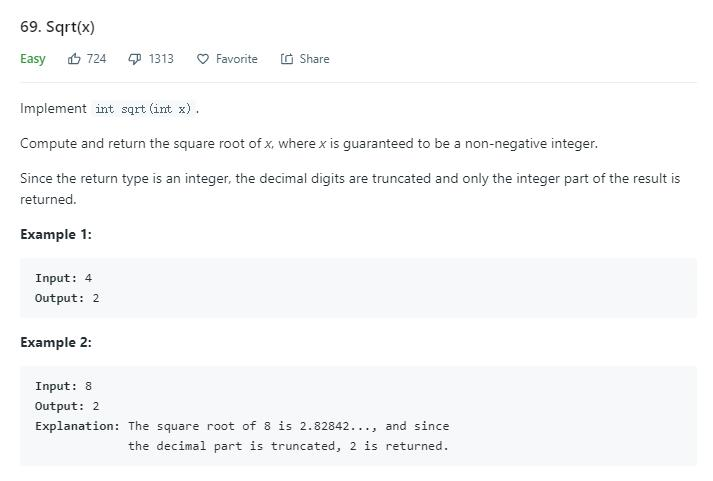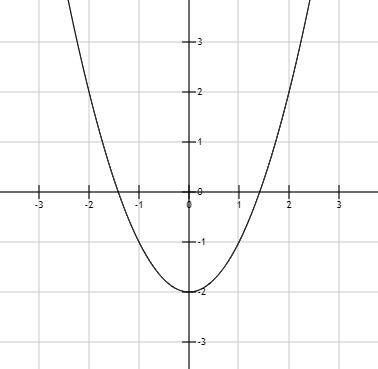# 题目描述（简单难度）# 解法一 二分法

public int mySqrt(int x) {
int L = 1, R = x;
while (L <= R) {
int mid = （L + R) / 2;
int square = mid * mid;
if (square == x) {
return mid;
} else if (square < x) {
L = mid + 1;
} else {
R = mid - 1;
}
}
return ?;
}


public int mySqrt(int x) {
int L = 1, R = x;
int ans = 0; //保存最后的解
while (L <= R) {
int mid = （L + R) / 2;
int square = mid * mid;
if (square == x) {
return mid;
} else if (square < x) {
ans = mid; //存起来以便返回
L = mid + 1;
} else {
R = mid - 1;
}
}
return ans;
}


int mid = （L + R) / 2;
int square = mid * mid;


int mid = L + (R - L) / 2;
int div = x / mid;


else if (square < x)
mid * mid < x
mid < x / mid
mid < div


public int mySqrt(int x) {
int L = 1, R = x;
int ans = 0;
while (L <= R) {
int mid = L + (R - L) / 2;
int div = x / mid;
if (div == mid) {
return mid;
} else if (mid < div) {
ans = mid;
L = mid + 1;
} else {
R = mid - 1;
}
}
return ans;
}


# 解法二 二分法求精确解int ans = (int) Math.round(mid); //先采取四舍五入
if ((long) ans * ans > n) {
ans--;
}
// 可以不用 long
if (ans > n / ans) {
ans--;
}


//计算 x² - n
public double fx(double x, double n) {
return x * x - n;
}

public int mySqrt(int n) {
double low = 0;
double high = n;
double mid = (low + high) / 2;
//函数值小于 0.1 的时候结束
while (Math.abs(fx(mid, n)) > 0.1) {
//左端点的函数值
double low_f = fx(low, n);
//中间节点的函数值
double low_mid = fx(mid, n);
//判断哪一段的函数值是异号的
if (low_f * low_mid < 0) {
high = mid;
} else {
low = mid;
}
mid = (low + high) / 2;
}
//先进行四舍五入
int ans = (int) Math.round(mid);
if (ans != 0 && ans > n / ans) {
ans--;
}
return ans;
}


# 解法三 牛顿法

$x_{k+1}=x_k- f(x_k)/f^{'}(x_k)$

$x_{k+1}=x_k-(x_k^2-n)/2x_k=(x_k^2+n)/2x_k = (x_k + n /x_k)/2$

public int mySqrt(int n) {
double t = n; // 赋一个初值
while (Math.abs(t * t - n) > 0.1) {
t = (n / t + t) / 2.0;
}
//先进行四舍五入
int ans = (int) Math.round(t);
//判断是否超出
if ((long) ans * ans > n) {
ans--;
}
return ans;
}